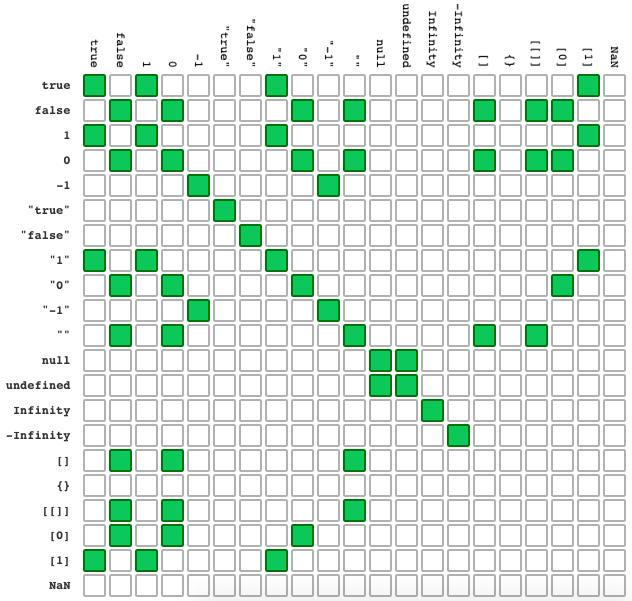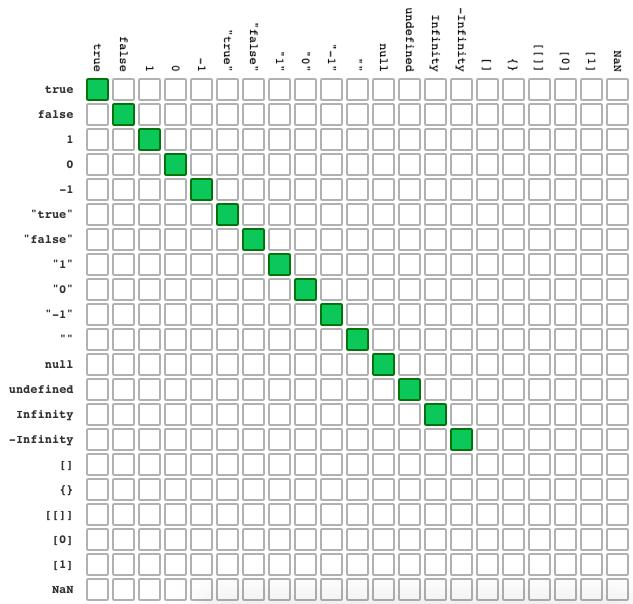# Difference between == and === in JavaScript - Full explanation

Don't know the difference between the various JavaScript comparison operators? When to use ==, ===, != or !==? Read this article and you will have all the information you need.

JavaScript has two ways to compare values. One way is more accurate (===), while the other has also a type conversion (==). Example: 1 == '1' (true). 1 === '1' (false).

Although it is a simple concept, understanding some details about JavaScript comparisons can save you a lot of time.

## Comparison operators: == vs ===

In JavaScript we have two very similar comparison operators, but in practice they provide very different results.

When we use three equal signs ===, it's performed what is called a "strict comparison". First, the type of values in question are compared, and then the actual values are compared.

When two equal signs are used ==, an abstract comparison is done. First, an attempt is made to convert the values to the same type and then the comparison is carried out.

See the example below that illustrates each type of comparison and its results.

``````console.log(1 === 1); // true
console.log(1 === '1'); // false

console.log(1 == 1); // true
console.log(1 == '1'); // true
``````

Operation 1 === '1' returns false, because the type of the first value is numeric, while the second is a string, so it is not necessary to compare the values and the process can return false right away because in the strict comparison it's necessary that both the value and the type of the variables are equal in order to return true.

The operation 1 == '1' returns true because when we make an abstract comparison, a type conversion will be performed beforehand. In this case, the conversion from string to a number is done and finally, the comparison of values is done where the result is true.

In theory, the strict comparison tends to be faster, because it doesn't need to convert the values, and in case they are of different types, it returns false right away. But the difference between the two methods of comparison is so insignificant that this should not be the reason to want to use one or the other.

So far, so good, here are other similar examples:

``````// First, a conversion is performed, then the comparison is performed
console.log(22 == '22'); // true

// Different types (int and string)
console.log(22 === '22'); // false

// Equal types, different values
console.log(22 === 11); // false

// Type and value are equal
const a = 22;
console.log(a === 22); // true
``````

Now take a look at not so obvious examples:

``````// Different types
console.log(1 === true); // false
console.log(0 === false); // false

// After conversion, values are the same
console.log(1 == true); // true
console.log(0 == false); // true
``````

Note that zeros and ones can represent true and false in an abstract comparison. And not only these values, but other values like undefined and null can represent false in JavaScript, so it's useful to remember some caveats.

## Some caveats about JavaScript comparisons

Understanding these caveats can save you a lot of time in debugging a code that doesn't work. Especially in JavaScript, where some operations can return values like `NaN`, `undefined`, `null`, etc.

Imagine a scenario where you need to check if the result of an input calculation has the value "0", but for some reason, you've made a mistake and initialized the variable with an empty value, so it will have the undefined value:

``````var input;
// ...
if (input == 0) { // undefined == 0 - true
// The code will be executed as if the input is equal to 0, but in fact it's undefined
}
``````

Using an abstract comparison in this case while not knowing about other values that may represent false can make you waste hours trying to figure out the error.

See the examples below to understand better:

``````// NaN is not equal to anything, not even itself
NaN === NaN; // false

// null and false when converted to a common type, are basically the same thing
null == false; // true

// undefined and false when converted to a common type, are basically the same thing
undefined == false; // true

// undefined and null are values that are not boolean, but can represent false in a comparison if (undefined) or if (null)
undefined == null; // true

// Just like null and undefined, an empty string can also represent false
'' == false; // true

// An empty array also represents false in the abstract comparison, but when compared to itself in an if, it returns true
[] == false; // true
``````

See below all the values that can represent false in JavaScript:

• `false` - Boolean value.
• `'', "" or ``` - All kinds of empty string.
• `0` - The number zero, even with a negative sign, represents false.
• `null`
• `undefined`
• `NaN` - Not a number.
• `[]` - Empty Array.

Remember that when we compare complex types of variables such as arrays or objects, the results are not based on the content of the object, but on where these objects point to in memory. Example:

``````const obj = { a: 1 };
const obj2 = obj;

// The variable obj and the object instantiated { a: 1 } are different objects, because they point to different addresses in the computer memory
console.log(obj == { a: 1 }); // false

// When obj2 is instantiated, it receives the same address of obj, therefore having the same value, even in strict comparison
console.log(obj === obj2); // true
``````

## Comparison operators !== vs !===

In the same way as operators of abstract or strict equality, we can also check whether two values are different in a strict or abstract manner.

Examples:

``````// First, it's performed a type conversion, then it checks if both values are different
console.log(1 != '1'); // false

// The types being different, the result is true, therefore the whole operation is true
console.log(1 !== '1'); // true
``````

### Conclusion

As you may have noticed, unless you already have a good familiarity with JavaScript, comparisons and value types can be a bit confusing at first, especially for those types that can represent false.

Understanding the results of the main abstract comparisons can be of great help, but in general, it's recommended to always use the operator of triple equals, so you can make sure that you are making a complete comparison.

See the table below that represents the use of two equals and see how things can get confusing:Agora veja o quão mais fácil é o uso de três iguais:Finally, I leave the link for the full comparison table and paraphrase the message from its author: "Punch line: Always use 3 equals, unless you have a good reason to use 2".